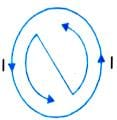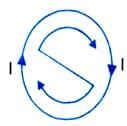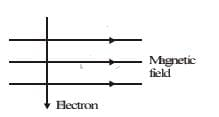# Test : Magnetic Effects of Current- 3

## 25 Questions MCQ Test NCERT Textbooks (Class 6 to Class 12) | Test : Magnetic Effects of Current- 3

Description
Attempt Test : Magnetic Effects of Current- 3 | 25 questions in 25 minutes | Mock test for UPSC preparation | Free important questions MCQ to study NCERT Textbooks (Class 6 to Class 12) for UPSC Exam | Download free PDF with solutions
QUESTION: 1

### The fact that magnetic field is produced around a wire carrying a current, was discovered by

Solution:

The correct answer is B as e electric current through the copper wire has produced a magnetic . In 1820 he accidentally discovered that a compass needle got deflected ... Through this observation Oersted showed that electricity and . representing the magnetic field around a current-carrying straight wire.

QUESTION: 2

### When the current is passing through the straight wire then, the associated magnetic field is

Solution:

The magnetic field around a straight current carrying conductor or wire can be increased by bending the wire into circular loop. A circular loop is made up of large number of very small straight wires.A magnetic field is produced by an electric current flowing through a circular coil of wire.Each small section of current carrying wire contributes to magnetic field lines. At the centre of circular wire,field lines become straight and perpendicular to the plane of coil.Right hand thumb rule is used to determine the direction of magnetic field produced due to current carrying circular wire.

QUESTION: 3

### When current is circular, the associated magnetic field is

Solution:

When current flows through a straight conductor, it is said that the current is straight. The magnetic field produced due to a straight current carrying conductor is in the form of concentric circles. The centre of the circles lies on the conductor itself and are in the plane perpendicular to the straight conductor. This implies that the magnetic field produced due to straight current is circular.

QUESTION: 4

When current flows clockwise in a loop, the polarity of its face is

Solution:

Clock rule states that if the current at any face of the loop flows in clockwise direction, that face behaves like a south pole. And, if the current round any face of the loop is in anti-clockwise direction, that face behaves like a north pole.QUESTION: 5

When current flows anticlockwise in a loop the magnetic polarity of the face is

Solution:

The polarity of this magnet can be understood with the help of clock face rule. If the current is flowing in anti-clockwise direction, then the face of the loop shows North pole.

QUESTION: 6

For a solenoid carrying a current I and having n turns per unit length, wrapped on a core of permeability, the correct expression for magnetic field intensity (2) is ?

Solution:

A simple electromagnet can be created by wrapping a coil of wire around a soft iron  The magnetic field strength of an electromagnet is therefore determined by the  turns of wire in the coil the greater will be the strength of the magnetic field.

QUESTION: 7

Magnets having temporary magnetism are called

Solution:
QUESTION: 8

Direction of force acting on a current carrying conductor kept in a magnetic field is given by

Solution:
QUESTION: 9

The electric device which works on the phenomenon of force on a current carrying conductor in a magnetic field is

Solution:
QUESTION: 10

Electromagnetic induction was discovered by

Solution:
QUESTION: 11

Direction of induced current produced by motion of a conductor in a magnetic field is given by

Solution:

In an electric generator mechanical energy is used to rotate a conductor in a magnetic field to produce electricity. Fleming's right hand rule is used where motion and magnetic field is given and the current is induced.

QUESTION: 12

In domestic electric circuits, fuse must be placed in series with

Solution:

The fuse wire is always connected in the live wire of the circuit because if the fuse is put in the neutral wire, then due to excessive flow of current when the fuse burns, current stops flowing in the circuit, but the appliance remains connected to the high potential point of supply through the live wire .

QUESTION: 13

Electric and main switch is contained in a main board fitted usually

Solution:

Electric and main switch is contained in a main board fitted usually in varandah or poarch . Because it will be safe there.

QUESTION: 14

High powered electrical appliances are earthed to

Solution:
QUESTION: 15

In a three pin socket (shoe) the bigger hole is connected to

Solution:

The bigger hole is connected to the earth wire and the other two holes are connected to the live wire and neutral wire respectively.

QUESTION: 16

Solution:
QUESTION: 17

Coming of live wire and neutral wire in direct contact causes

Solution:

When live wire comes in direct contact with the neutral wire the very high current flows through the circuit producing a large amount of heat. This phenomenon is called the Short Circuit.

QUESTION: 18

Melting point of material of a fuse wire must be

Solution:
QUESTION: 19

A high powered electric appliance has used inferior wires and is not earthed. It is a source of

Solution:
QUESTION: 20

Which of the following correctly describes the magnetic field near a long straight wire ?

Solution:

The field consists of concentric circles centred on the wire.
On applying right-hand thumb rule, we find the direction of magnetic field. The field is in the form of concentric circles centred on the wire carrying current.
Hence, the option (d) is correct.

QUESTION: 21

The phenomenan of electromagnetic induction is

Solution:
QUESTION: 22

The device used for producing electric current is called a

Solution:
QUESTION: 23

At the time of short-circuit, the current in the circuit

Solution:
QUESTION: 24

An electron enters a magnetic field at right angles to it as shown in figure. The direction of force acting on the electron will beSolution:

According to Fleming's Left Hand rule, the direction of force is perpendicular to the direction of magnetic field and current. Here, since the direction of current is taken opposite to the direction of motion of electrons, the force is directed into the page.

*Multiple options can be correct
QUESTION: 25

Which of the following property of a proton can change while it moves freely in a magnetic field? (There may be more then one correct answers)

Solution:

The correct answers are (c) and (d). Each moving charged particle in a magnetic field experiences a force. The direction of force experienced by a positive charge (i.e., a proton) is given by Fleming's left hand rule. The force acting on the proton would change both velocity and momentum.Use Code STAYHOME200 and get INR 200 additional OFF Use Coupon Code